natanael.arndt.xyz

Introduction

The Semantic Web Layer Cake, Semantic Web Layer Model, or Semantic Web Stack is an approach to define and visualize the interplay of the components on the Semantic Web. The most commonly known layer model probably is the OSI model. The OSI model describes which tasks are performed in which layer and thus provides an abstraction of these tasks for the other layers. In this way it is not necessary for every single application to implement the full stack. Further it allows to make changes within one layer by keeping applications on the other layers in tact.

Since abstraction simplifies aspects this is usually a good point for discussion. Also the Semantic Web Layer Cake is permanently under discussion. To keep an overview on the individual proposals I want to keep a collection of links to the individual figures. If you know more examples or of you can give me “more original” sources, please tell me.

The Commonly Used Layer Cake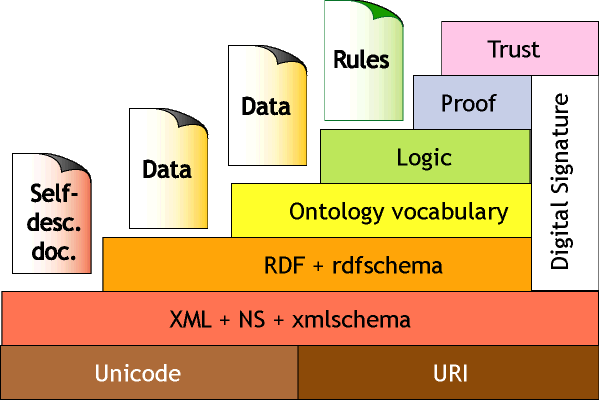The first widely used and referenced model was presented by Tim Berners-Lee in 2000.

Based on the 2000 version of the model a model was proposed in 2005-2007 which is widely used with small variations:This one is referenced in the MIT-W3C DAML program: Final Report, in Semantic Web Technologies in the Enterprise by Lee Feigenbaum on November 28, 2006 and in the talks Emerging Web Technologies to Watch by Steve Bratt. All around the same time, so I don’t know who created it. (Alternative URL of the figure and also an SVG version in the Wikipedia.)In the talks Semantic Web, and Other Technologies to Watch by Steve Bratt from 2007 he is using a different version.

Alternative Proposals and InterpretationsIn the article The Semantic Web - Not a piece of cake… by Benjamin Nowack from 2009, he puts some more dimensions into the layer model which provides nice angles to view on the things. He also provides Linked Data with an extra part of the Stack. A slightly modified version was published together with a link collection in The common, layered Semantic Web technology stack by Thomas Gängler in 2011.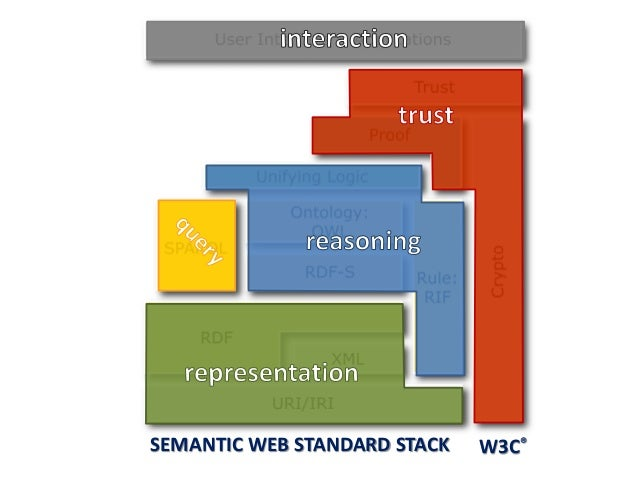In the presentation An introduction to Semantic Web and Linked Data by Fabian Gandon at the WWW2014, he categorizes the layers into the five groups: Abstract Language/Representation, Query & Update, Reasoning, Trust, and Application and Interaction.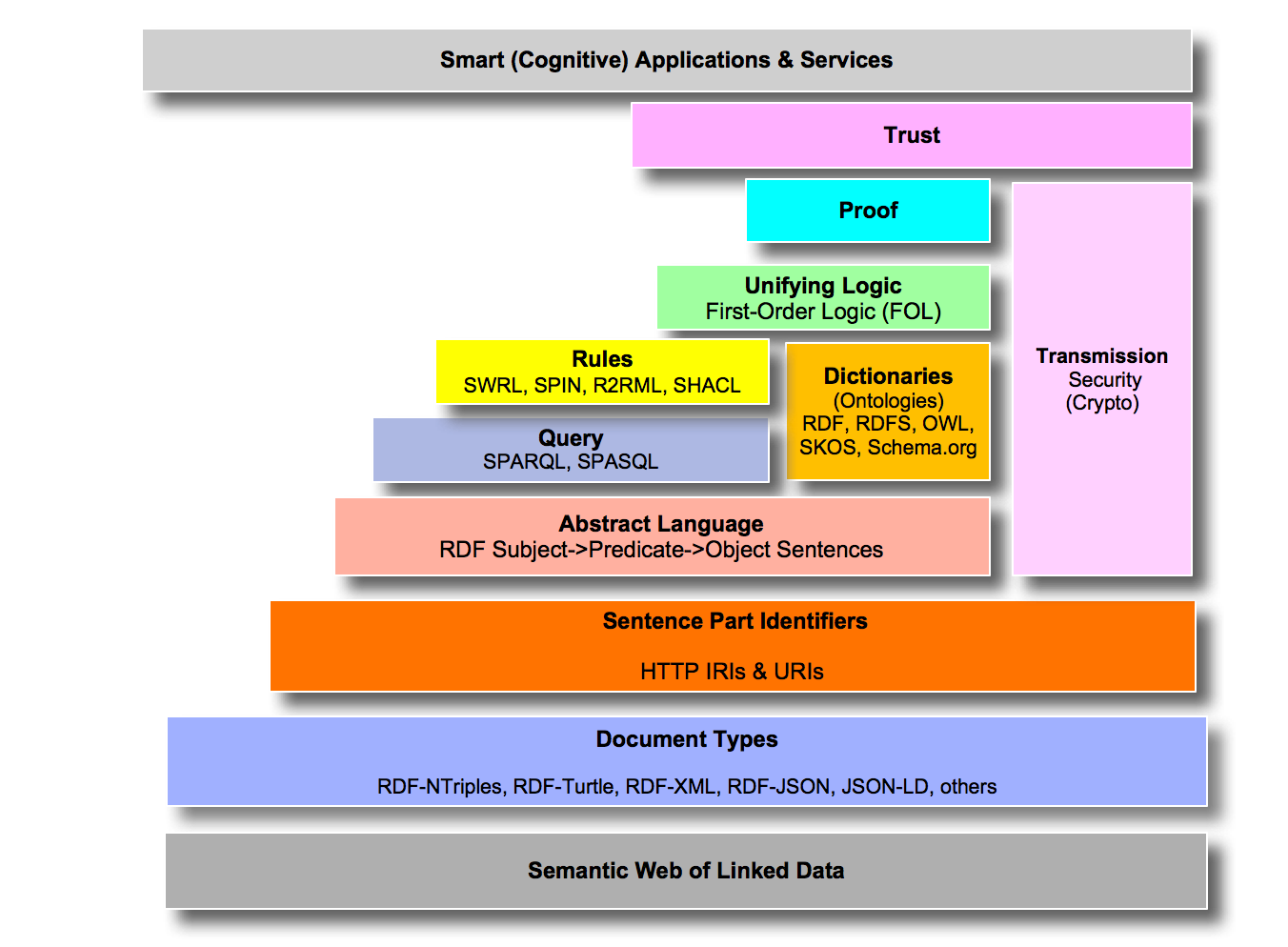In the article Semantic Web Layer Cake Tweak, Explained by Kingsley Uyi Idehen from 2017, he shows an updated model which includes the recent evolution of the Semantic Web technologies.

Historical Models

The first widely used and referenced model by Tim Berners-Lee was also predated by some other models.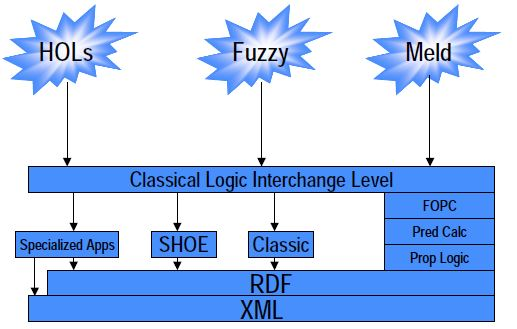by Jim Hendler from 2000 (via Semantics for Interoperable Systems by John Sowa)

John Sowa also shows this figure in his page: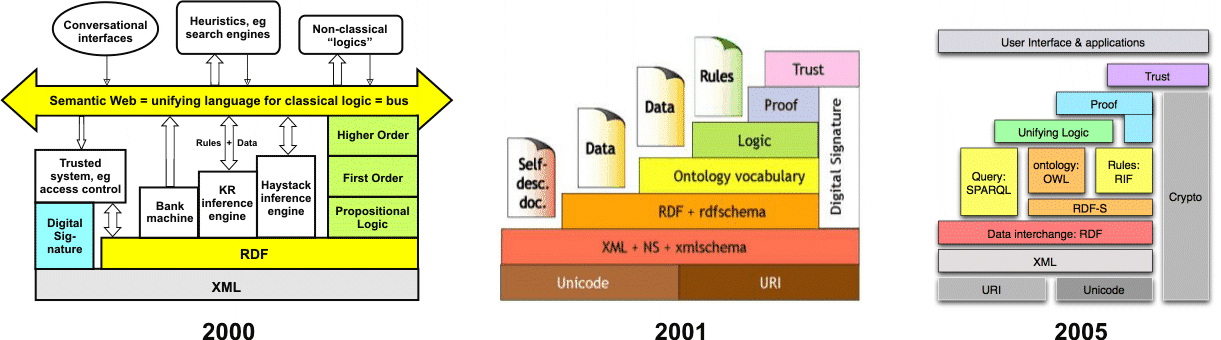TikZ Version

I was looking for a TikZ version of the layer cake but coudn’t find one, so here it is:

\documentclass[tikz]{standalone}
\usepackage{tikz}

% Semantic Web Layer Cake: https://www.w3.org/2007/03/layerCake.svg
% TikZ interpretation by Natanael Arndt <arndtn@gmail.com>
% If you like: CC-by
% If you don't want to place a name: CC-0

\begin{document}

\tikzstyle{label}=[align=center]
\tikzstyle{layer}=[draw, label, anchor=south west, minimum height=.75cm, minimum width=1cm]

\begin{tikzpicture}[]
%\draw[help lines,step=.25,color=gray!20] (0,0) grid (9,8.75);

% Representation
\draw (0,0) node    (iri)     [layer, minimum width=7.75cm]           {URI/IRI\strut};
\draw (4,1) node    (xml)     [layer, minimum width=3.75cm]           {XML\strut};
\draw (0,1) -- ++(0,1.75) -- ++(7.75,0) -- ++(0,-.75) -- ++(-4,0) -- ++(0,-1) -- cycle;
\node at (2,2)                [anchor=base, text width=3.5cm, label]  {Data interchange:\\RDF\strut};

% Query & Update
\draw (.5,3) node   (query)   [layer, minimum width=1.75cm, minimum height=1.75cm, text width=1.5cm] {Query: SPARQL\strut};

% Reasoning
\draw (2.5,3) node  (rdfs)    [layer, minimum width=3.25cm]           {RDFS\strut};
\draw (2.5,4) node  (owl)     [layer, minimum width=3.25cm]           {Ontology: OWL\strut};
\draw (6,3) node    (rif)     [layer, minimum width=1.75cm, minimum height=1.75cm, text width=1.5cm] {Rule: RIF\strut};
\draw (1.5,5) node  (logic)   [layer, minimum width=5.25cm]           {Unifying Logic\strut};

% Trust
\draw (4,6) -- ++(0,.75) -- ++(3.75,0) -- ++(0,-1.75) -- ++(-.75,0) -- ++(0,1) -- cycle;
\node at (6,6.25)             [anchor=base, text width=3.5cm, label]  {Proof\strut};
\draw (8,0) node    (crypto)  [layer, minimum height=6.75cm]          {};
\node at (8.5,3.5)            [label, rotate=90]                      {Crypto\strut};
\draw (5.25,7) node (trust)   [layer, minimum width=3.75cm]           {Trust\strut};

% Interaction
\draw (0,8) node    (ui)      [layer, minimum width=9cm]              {User Interface \& Applications\strut};
\end{tikzpicture}
\end{document}

[PDF], [TeX+TikZ]